# How to Calculate and Solve for Van’t Hoff Factor, Ebullioscopic Constant, Molality and Boiling Point Elevation | The Calculator Encyclopedia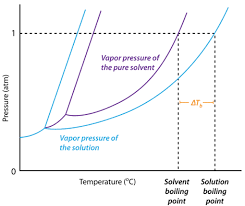The image above represents boiling point elevation.

To compute for the boiling point elevation, three parameters are needed and these parameters are Van’t Hoff’s Factor (i), ebullioscopic constant (Kb) and Molality.

The formula for calculating boiling point elevation:

δTb = iKb x Molality

Where;
δTb = boiling point elevation
i = Van’t Hoff’s Factor
Kb = ebullioscopic constant
Molality

Let’s solve an example;
Find the boiling point elevation when the Van’t Hoff’s Factor is 42, ebullioscopic constant is 60 and molality of 180.

This implies that;
i = Van’t Hoff’s Factor = 42
Kb = ebullioscopic constant = 60
Molality = 180

δTb = iKb x Molality
δTb = (42)(60) x 180
δTb = (2520) x 180
δTb = 453600

Therefore, the boiling point elevation is 453600 °C m-1.

Calculating the Molality using the Boiling Point Elevation, Van’t Hoff’s Factor and Ebullioscopic Constant.

Molality = δTb / iKb

Where;
Molality
δTb = boiling point elevation
i = Van’t Hoff’s Factor
Kb = ebullioscopic constant

Let’s solve an example;
Find the molality with a boiling point elevation of 120 and a van’t hoff’s factor of 32 with a ebullioscopic constant of 12.

This implies that;
δTb = boiling point elevation = 120
i = Van’t Hoff’s Factor = 32
Kb = ebullioscopic constant = 12

Molality = δTb / iKb
Molality = 120 / 384
Molality = 0.3125

Therefore, the molality is 0.3125.

Calculating the Van’t Hoff’s Factor when the Boiling Point elevation, Ebullioscopic Constant and Molality is Given.

i = δTb / Kb x molality

Where;
i = Van’t Hoff’s Factor
δTb = boiling point elevation
Kb = ebullioscopic constant
Molality

Let’s solve an example;
Find the van’t hoff’s factor when the boiling point elevation is 240 with a molality of 10 and an ebullioscopic constant of 15.

This implies that;
δTb = boiling point elevation = 240
Kb = ebullioscopic constant = 15
Molality = 10

i = δTb / Kb x molality
i = 240 / 15 x 10
i = 240 / 150
i = 1.6

Therefore, the Van’t Hoff’s Factor is 1.6.

Calculating the Ebullioscopic Constant when Boiling Point Elevation, Molality and Van’t Hoff’s Factor is Given.

kb = δTb / i x molality

Where;
Kb = ebullioscopic constant
i = Van’t Hoff’s Factor
δTb = boiling point elevation
Molality

Let’s solve an example;
Find the ebullioscopic constant when the boiling point elevation is 180 with a molality of 8 and a van’t hoff’s factor of 15.

This implies that;
δTb = boiling point elevation = 180
i = Van’t Hoff’s Factor = 15
Molality = 8

kb = δTb / i x molality
kb = 180 / 15 x 8
kb = 180 / 120
kb = 1.5

Therefore, the ebullioscopic constant is 1.5.

Nickzom Calculator – The Calculator Encyclopedia is capable of calculating the boiling point elevation.

To get the answer and workings of the boiling point elevation using the Nickzom Calculator – The Calculator Encyclopedia. First, you need to obtain the app.

You can get this app via any of these means:

To get access to the professional version via web, you need to register and subscribe for NGN 1,500 per annum to have utter access to all functionalities.
You can also try the demo version via https://www.nickzom.org/calculator

Once, you have obtained the calculator encyclopedia app, proceed to the Calculator Map, then click on Basic Chemistry under Chemistry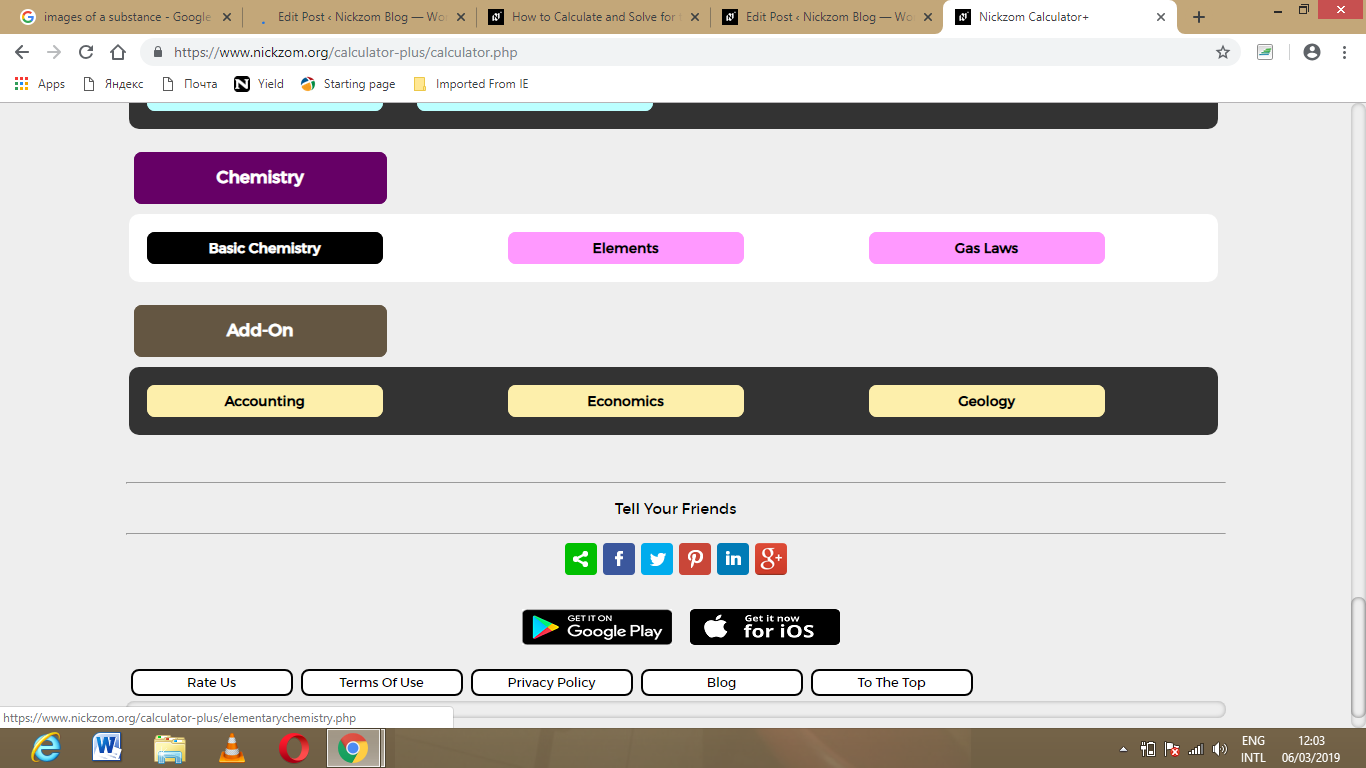Now, Click on Boiling Point Elevation under Basic Chemistry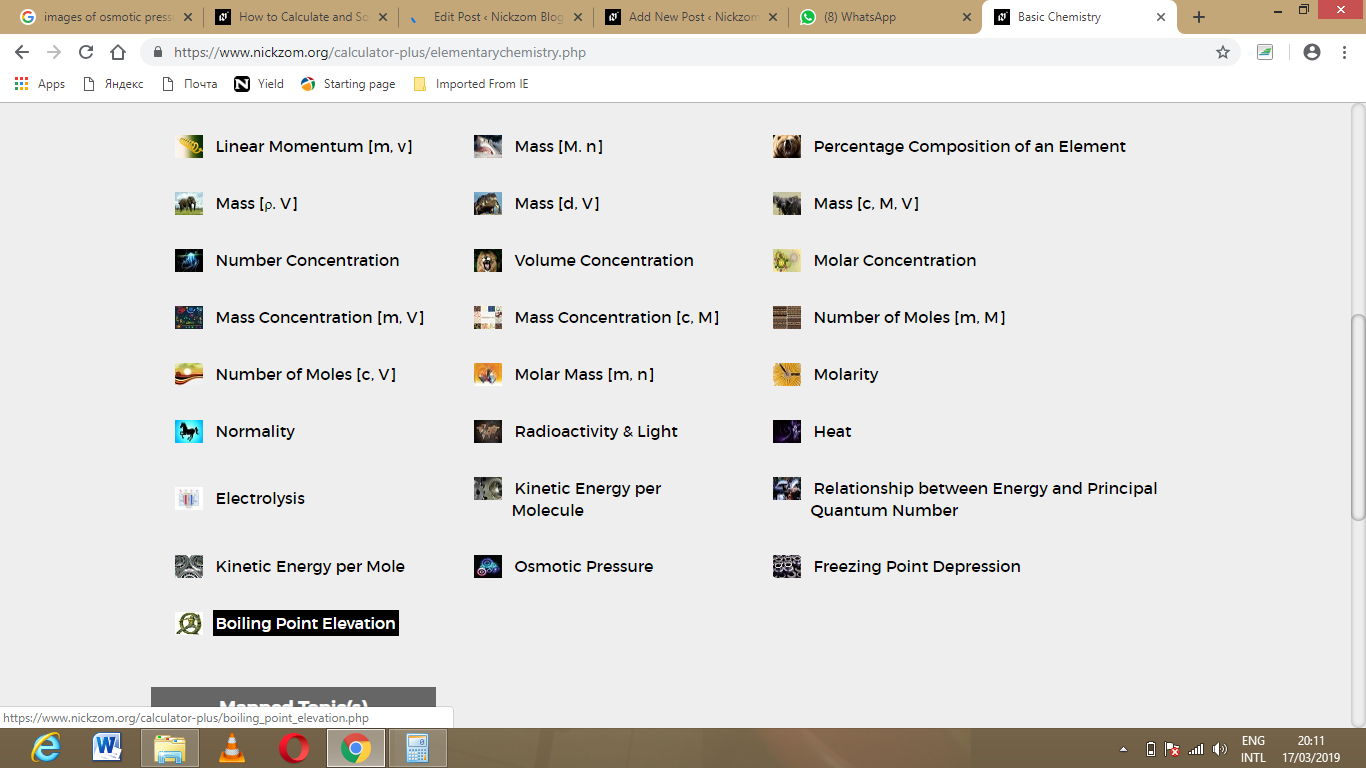The screenshot below displays the page or activity to enter your values, to get the answer for the boiling point elevation according to the respective parameters which are the Van’t Hoff’s Factor (i), Ebullioscopic Constant (Kb) and Molality.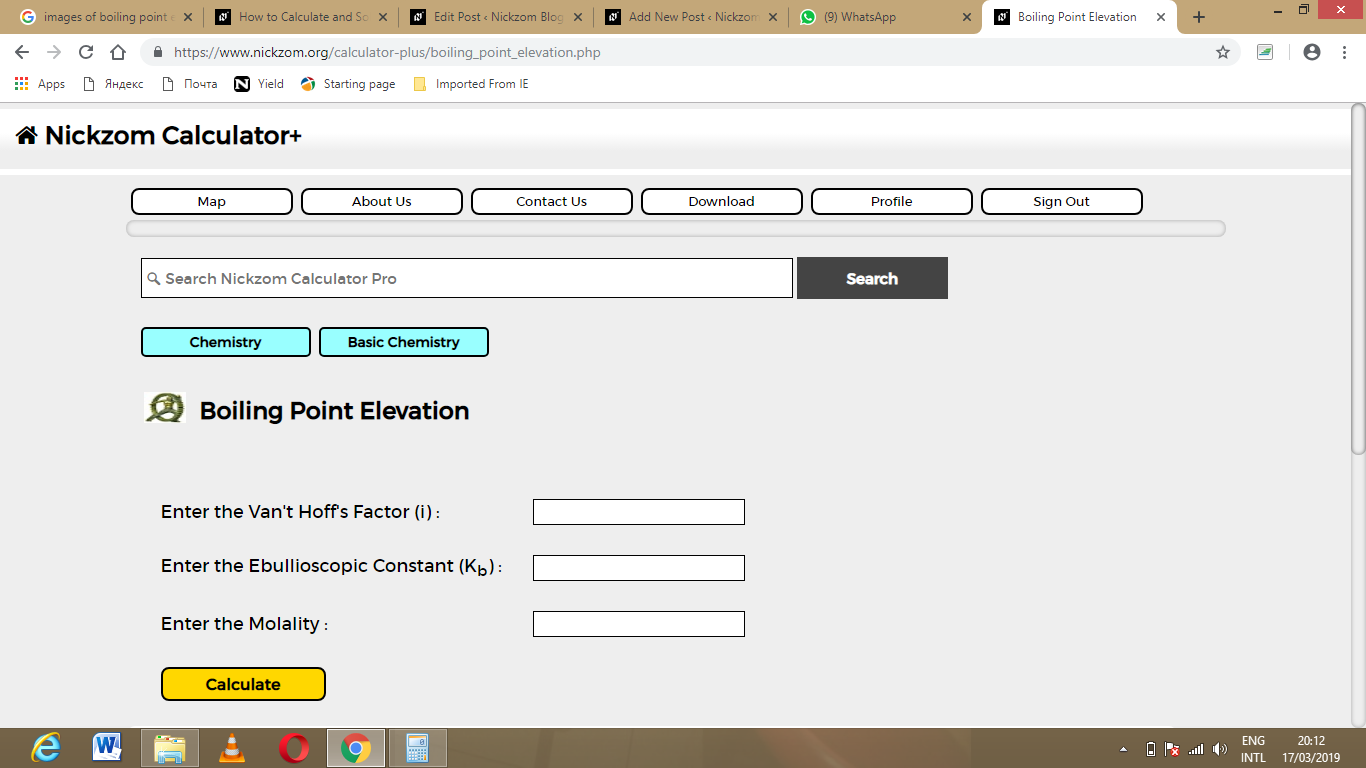Now, enter the values appropriately and accordingly for the parameters as required by the example above where the Van’t Hoff’s Factor (i) is 42, Ebullioscopic Constant (Kb) is 60 and Molality is 180.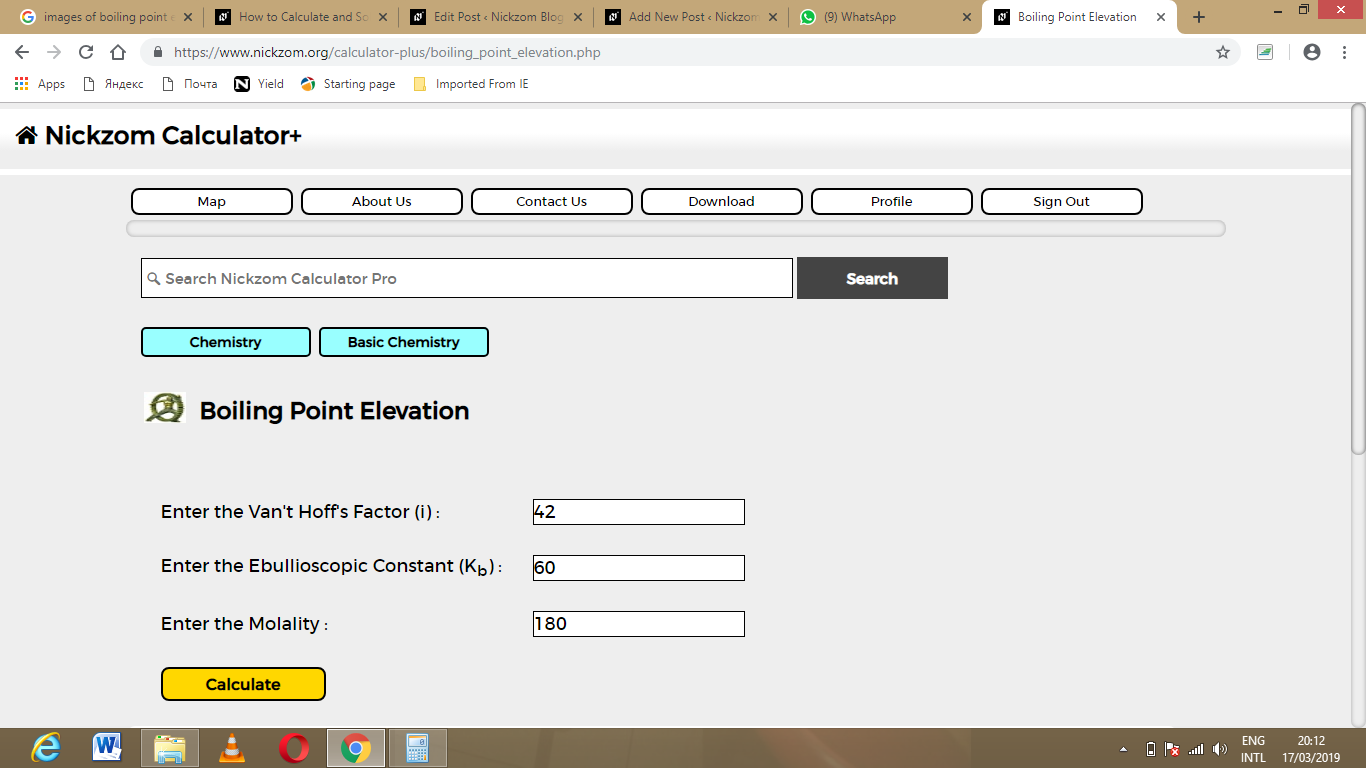Finally, Click on Calculate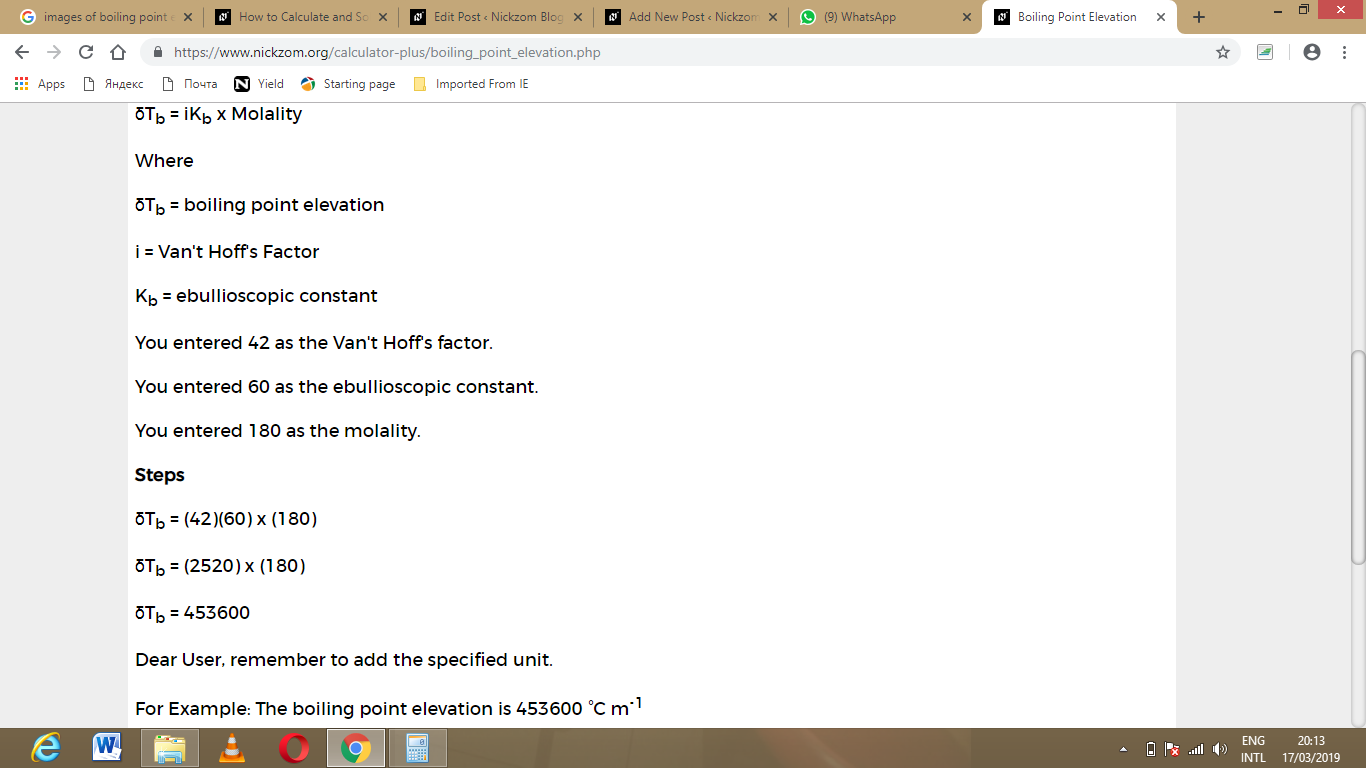As you can see from the screenshot above, Nickzom Calculator – The Calculator Encyclopedia solves for the boiling point elevation and presents the formula, workings and steps too.